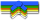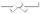# Search Results

Searching for: if

## Individual Exercises

0 / 50

Given a string, does "xyz" appear in the middle of the string? To define middle, we'll say that the number of chars to the left and right...0 / 50

Given a day of the week encoded as 0 = Sun, 1 = Mon, 2 = Tue, ... 6 = Sat, and a boolean indicating if we are on vacation, return a...0 / 50

The number 6 is a truly great number. Given two `int` values, `a` and `b`, return true if either one is 6. Or if their sum or difference is 6....0 / 50

When squirrels get together for a party, they like to have cigars. A squirrel party is successful when the number of cigars is between 40...0 / 50

You and your date are trying to get a table at a restaurant. The parameter `you` is the stylishness of your clothes, in the range 0..10,...0 / 50

You have a green lottery ticket, with `int`s `a`, `b`, and `c` on it. If the numbers are all different from each other, the result is 0. If all...0 / 50

Write a method called `listSearch()` that takes in a target string and a list of other strings. This method returns a (possibly shorter)...0 / 50

Write an `ArrayBasedStack<T>` member method called `reverseString()` that takes a String as a parameter, then uses an ArrayBasedStack...0 / 50

Write an `ArrayBasedStack<T>` member method called `decimalToBinary()` that takes a decimal as an int parameter, then uses an...0 / 50

Write a function in Java that implements the following logic: Given a string, if the string begins with "red" or "blue" return that color...0 / 70

Given an integer array length of 1 or more, return the difference between the largest and smallest values in the array. Note that the...0 / 50

Return an array that is "left shifted" by one--so for an input of {6, 2, 5, 3}, the method returns {2, 5, 3, 6}. You may modify and...0 / 10

Given a binary tree, write a recursive function to return the difference between the sum of all node values at odd levels and sum of all...0 / 10

Write a recursive function `BSTsmallcount` that, given a BST and a value `key`, returns the number of nodes having values less than `key`. Your...0 / 10

Write a recursive function `BSTsmallcount` that, given a BST and a value `key`, returns the number of nodes having values less than `key`. Your...0 / 10

Given a binary tree, check if the tree satisfies the property that for each node, the sum of the values of its left and right children...0 / 10

Write a recursive function that returns true if there is a node in the given binary tree with the given value, and false otherwise. Note...0 / 10

Given a binary tree, write a recursive function to return the difference between the sum of all node values at odd levels and sum of all...0 / 10

The height of a binary tree is the length of the path to the deepest node. An empty tree has a height of 0, a tree with one node has a...0 / 10

Write a recursive function that increments by one the value for every node in the binary tree pointed at by `root`, then returns the...0 / 10

Write a recursive function to count the number of leaf nodes in the binary tree pointed at by `root`. You must use the `isLeaf` method to...0 / 10

We define a "root-to-leaf path" to be any sequence of nodes in a tree starting with the root node and proceeding downward to a leaf. The...0 / 10

Write a recursive function to set the value for each node in a binary tree to be its depth then return the modified tree. Assume that...0 / 10

Write a recursive function that returns the sum of the values for all of the nodes of the binary tree with root `root`.
Here are methods...0 / 10

Given two binary trees, return true if and only if they are mirror images of each other. Note that two empty trees are considered mirror...